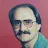APP Information

 Download Version 1.2 (5) Apk Size 5.06 MB App Developer Math Apps Malware Check TRUSTED Install on Android 4.1.x and up App Package com.mathapps.volumeareacalculator.apk MD5 fd6d069fb663b6751de1165cc1cf9a95 Rate 5### App Description

```Area Calculator: You are able to calculate the area for the most important geometric figures. You can calculate the area of circle, ellipse, rectangle, square, trapezoid, triangle, parallelogram, rhombus, sector, geometrical shapes, two dimensional, three dimensional and triangle.

Volume Calculator: You are able to calculate the volume for the most important geometric objects. You can calculate the volume of cone, cube, cuboid, cylinder, sphere, square pyramid and tetrahedron.

Very basic as well as complex formulas are listed. The image of object is also displayed for better clarity in understanding.

Best math tool for school and college! If you are a student, it will help you to learn geometry plus Area volume and surface area formulas.!

Highly useful for students.
►App showcases perimeter and area formulas for 2D objects, and surface area & volume
formulas for 3D objects.
►You can calculate the area of circle, area of ellipse, area of rectangle, area of square, area of trapezoid, area of triangle, area of parallelogram, area of rhombus, area of sector, area of geometrical shapes, area of two dimensional, area of three dimensional and area of triangle.
►You can calculate the volume of cone, volume of cube, volume of cuboid, volume of cylinder, volume of sphere, volume of square pyramid and volume of tetrahedron.
►Other Calculations - HCF ,LCM , Average, Coordinate geometry, Square toot, Cube root, Exponent law, Simple interest etc..

Formulas for following type of geometrical objects are listed in this app.
Circle, Cone, Cube, Cuboid, Ellipse, Ellipsoid, Frustum, Kite, Parallelogram, Parallelepiped, Regular Polygon, Rectangle, Rectangular Prism, Rhombus, Sector, Sphere, Square, Torus, Trapezoid, Triangle.

List of plane and solid figures contained in the application:

Planimetry ( 2D Geometry ):
- Square
- Rectangle
- Parallelogram
- Trapezoid
- Scalene triangle
- Isosceles triangle
- Equilateral triangle
- Right triangle
- Simple polygon
- Regular convex polygon
- Circle / Disk
- Annulus
- Annular sector
- Circular sector
- Circular segment
- Ellipse
- Ellipse segment
- Cubic function
- Intercept theorem
- Kite
- Angles and Trigonometry
- Rhombus
- Incircle and circumcircle of a triangle

Stereometry ( 3D Geometry ):
- Cube
- Cuboid
- Right prism
- Oblique prism
- Right circular cylinder
- Oblique circular cylinder
- Cylindrical segment
- Cylindrical wedge
- Pyramid
- Frustum
- Obelisk
- Prismatoid
- Right circular cone
- Oblique circular cone
- Right truncated cone
- Oblique truncated cone
- Elliptic cone
- Truncated elliptic cone
- Sphere / Disk
- Spherical sector
- Sphere cap
- Spherical segment
- Ellipsoid
- Paraboloid of revolution
- Toroid
- Torus
- Right hollow cylinder
- Rectangular pipe
- Prism with a regular base
- Pyramid with a regular base
- Elliptical Cylinder
- Spherical Wedge

►Let us know if you have any suggestions/issues. If you think any other formula should be listed, then do write to us at [email protected]
```

### More Android Apps to Consider

1.James Bond

This is very interesting and amazing app. Very useful and helpfull app for students to learn. Very simple and easy to use

2.Dr Dream

Area and volume is brilliant app.Overall good experience!!!good offers as well .95% accurate .

3.Gary visaya

Sadly to say, Area and volume calculator doesnt work with my tablet android. Uninstalling.

4.Tienie Cloete

Great App with all the formula

5.Momo Doll

Area Calculator: You are able to calculate the area for the most important geometric figures. You can calculate the area of circle, ellipse, rectangle, square, trapezoid, triangle, parallelogram, rhombus, sector, geometrical shapes, two dimensional, three dimensional and triangle.

6.Area and Volume is outstanding and wonderful application. It has many features. I really love this application. I like this application very much

7.Area and volume is outstanding and wonderful application. It has many features. I really love this application. I like this application very much

8.Area and Volume calculator is a professional and a useful application of playstore. You can calculate total area of houses. I love this application.

9.Hunain Prince

Area and volume calculator is very interesting and amazing app . The app is very interesting because of the day by day famous and fantastic feature can be uploaded in this app. Amazing application.

10.sehrish khan

this is very useful and impressive area and volume calculator application great feature and superb quality one of my favourite application great efforts done by their developer I appreciate their hard work.